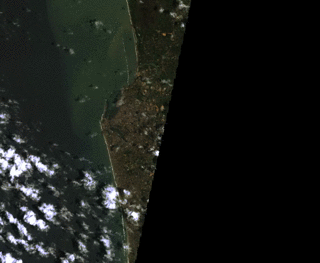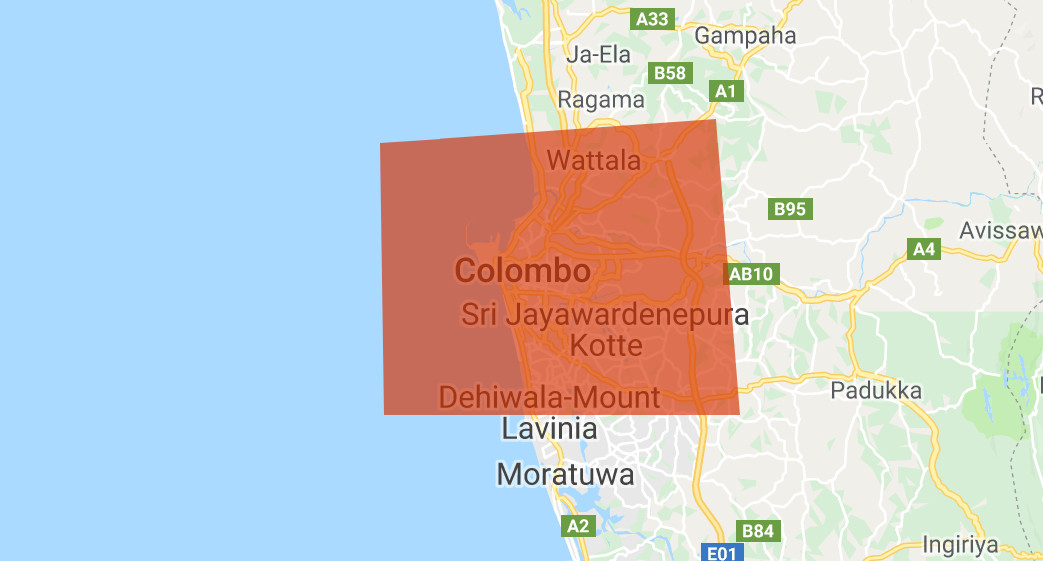# Timelapse movie

Timelapse movie from landsat 4, 5, 7 and 8

Step 1: Create a list containing all years

```// define the period
var years = ee.List.sequence(1988,2016,1);
```

Step 2: Create a list containing all years

```// import collections
var l4 = ee.ImageCollection("LANDSAT/LT04/C01/T1_SR");
var l5 = ee.ImageCollection("LANDSAT/LT05/C01/T1_SR");
var l7 = ee.ImageCollection("LANDSAT/LE07/C01/T1_SR");
var l8 = ee.ImageCollection("LANDSAT/LC08/C01/T1_SR");
```

Step 2: Create a list containing all years

```// import collections
var l4 = ee.ImageCollection("LANDSAT/LT04/C01/T1_SR");
var l5 = ee.ImageCollection("LANDSAT/LT05/C01/T1_SR");
var l7 = ee.ImageCollection("LANDSAT/LE07/C01/T1_SR");
var l8 = ee.ImageCollection("LANDSAT/LC08/C01/T1_SR");
```

Step 3: Set bandnames

```// set bandnames for rgb
var l4names = ee.List(["B1","B2","B3"]);
var l5names = ee.List(["B1","B2","B3"]);
var l7names = ee.List(["B1","B2","B3"]);
var l8names = ee.List(["B2","B3","B4"]);

// bands
var bands = ee.List(['blue','green','red']);
```

Step 4: Set bandnames

```// set bandnames for rgb
var l4names = ee.List(["B1","B2","B3"]);
var l5names = ee.List(["B1","B2","B3"]);
var l7names = ee.List(["B1","B2","B3"]);
var l8names = ee.List(["B2","B3","B4"]);

// bands
var bands = ee.List(['blue','green','red']);
```

Step 5: create a geometryStep 6: Filter for cloudcover and geometry:

```// Filter based on location
var l4images  = l4.filterBounds(geometry).filter(ee.Filter.lt("CLOUD_COVER",5));
var l5images  = l5.filterBounds(geometry).filter(ee.Filter.lt("CLOUD_COVER",5));
var l7images  = l7.filterBounds(geometry).filter(ee.Filter.lt("CLOUD_COVER",5));
var l8images  = l8.filterBounds(geometry).filter(ee.Filter.lt("CLOUD_COVER",5));
```

Step 7: Scale bands and select rgb

```function scale(image){
return image.multiply(0.0001).set("system:time_start",image.get("system:time_start"));
}

// Change the bandnames
l4images = l4images.map(scale).select(l4names,bands);
l5images = l5images.map(scale).select(l5names,bands);
l7images = l7images.map(scale).select(l7names,bands);
l8images = l8images.map(scale).select(l8names,bands);
```

Step 8: merge the collections into 1 collection

```var collection = l4images.merge(l5images).merge(l7images).merge(l8images).sort("system:time_start");

print(collection);
```

Step 9: merge the collections into 1 collection

```var collection = l4images.merge(l5images).merge(l7images).merge(l8images).sort("system:time_start");

print(collection);
```

Step 10: Create a 8-bit format and export the collections to video

```// we need an 8-bit format
var coll4Video = collection
.map(function(image) {
return image.multiply(512).uint8();   // need to make it 8-bit
});

// export the video to the drive
Export.video.toDrive({
collection: coll4Video.select(['red','green','blue']),
description: "Colombo" ,
scale: 30,
framesPerSecond: 4,
region: geometry.bounds()
});
```

1.Jo says:
1.Jo says: